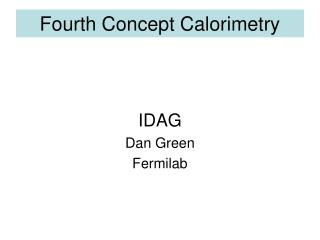# Fourth Concept Calorimetry - PowerPoint PPT PresentationDownload PresentationFourth Concept Calorimetry

Fourth Concept CalorimetryDownload Presentation## Fourth Concept Calorimetry

- - - - - - - - - - - - - - - - - - - - - - - - - - - E N D - - - - - - - - - - - - - - - - - - - - - - - - - - -
##### Presentation Transcript

1. Fourth Concept Calorimetry IDAG Dan Green Fermilab

2. DESY Review Previous calorimeter review was positive and supportive. Main issues are not technical but managerial.

3. Algebra for Dual Readout • Suppose both Q (quartz) and S (scint) are calibrated with an e beam, so Q and S are measured in “electron GeV”. • Suppose h/e is determined by mapping out pion/electron mean over a range of energy. • Where f = f(E) is the neutral energy fraction of the hadronic cascade. <f> evaluated a la Wigmans or Groom (PDG) – e.g. <f> = 0.11 log(E) • The aim of dual readout is to simultaneously measure E and f and thus avoid the resolution term due to neutral fluctuations event by event, dE = df(e-h) if the calorimeter is not compensating

4. Algebra - II • Measuring the Q and S response and knowing h/e for both Q and S, event by event E and f can be solved for: • The result for energy is linear in Q and S and therefore an ensemble of particles, a jet, can be measured and yield the correct energy, without df fluctuations, without the need to separate the energies of the individual hadrons in a jet as is needed in PF algorithms.

5. Algebra - III • On the other hand the result for f is non-linear, so that one gets a weighted mean for f. • For example 2 particle overlap in some cell:

6. Fourth Calor - Warsaw

7. Fourth @ Warsaw - Linearity Extracting E appears to be good to a few % w.r.t. the mean value.

8. Fourth UIC - Overall All fiber baseline. Depth is ~ 7.8 λ. Dual readout with time history (n)

9. Fourth- UIC, Energy Resolution Energy resolution achieved is within requirements

10. Fourth – UIC, n Detection The binding energy fluctuations are determined event by event using the delayed n recoils in the scint fibers. Note the anti-correlation of the n signal with the Q signal (e.m.)

11. Fourth – UIC, Particle ID Use S and C signals to separate mu (small energy deposit) and e (more C than S) and pions (mix of S and C)

12. Fourth – UIC, Crystals Baseline is fibers. Crystals have advantages. No longitudinal segmentation for redundant measurements.

13. Results from CMS Test Beams • For the LHC there have been extensive tests of the calorimetry • These data allow for the extraction of <fo>, the mean neutral fraction of a hadronic shower as a function of beam momentum for pion momenta between 2 and 300 GeV • There were TOF and Cerenkov available which made it possible to study responses to pi, K and p at low momenta.

14. Data on pion/electron Response This data was taken with a “mip” in the ECAL. The response is purely that of HCAL which is a brass/scintillator sandwich. Note that the data cannot be explained by a single logarithmic behavior for f.

15. Extraction of the Mean Neutral Fraction Assuming that for the HCAL, e/h = 1.4 independent of energy, one can extract the average neutral fraction as follows: Note that e/h=1.4 fits at high energy but fails, <fo> < 0 at low energies - < 10 GeV. If f>0 then pi/e > h/e=0.7

16. Particle Species Dependence The kaon and proton response is rather different from the pion response. In a single readout calorimeter this limits the resolution. In a dual readout it appears that a different f will be found at the same E for different species.

17. Depth of 7.8 Int Lengths Baseline is a fairly deep calorimeter with plastic (S) and quartz (Q) fibers. Depth is sufficient. Expect single particle resolution of: Where 35% -> 26% with slow n readout (binding energy losses) using time structure of S signal

18. Summary • The particle species dependence should be removed by solving for f event by event. For example f for pions will be larger than for p because the ‘leading particle’ can be a neutral pion in the former case, but a neutron in the latter. • Nevertheless, it would be useful to validate that assertion. • There appears to be energy dependence in the e/h ratios which appears at low energies. It would be useful for the Collaboration to log data below 20 GeV and then re-visit the calibration strategy. • In situ calibration of the HCAL if there is a crystal ECAL in front should be carefully examined.